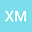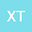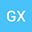Almost sharp global well-posedness for the defocusing Hartree equation with radial data in $\mathbb R^5$
•••• +1
• Xingjian Mu,
• Xingdong Tang,
• Guixiang Xu,
• Jianwei Yang
Xingjian Mu
Beijing Normal University
Author ProfileXingdong Tang
Nanjing University of Information Science and Technology
Author ProfileGuixiang Xu
Beijing Normal University
Author ProfileJianwei Yang
Beijing Institute of Technology
Author Profile## Abstract

We show global well-posedness and scattering for the defocusing, energy-subcritical Hartree equation \begin{equation*} iu_t + \Delta u =F(u), (t,x)\in\mathbb{R}\times\mathbb{R}^5 \end{equation*} where $F(u)= \big( V* |u|^2 \big) u$, $V(x)=|x|^{-\gamma}$, $3< \gamma< 4$, and initial data $u_0(x)$ is radial in almost sharp Sobolev space $H^{s}\left(\R^5\right)$ for $s>s_c=\gamma/2-1$. Main difficulty is the lack of the conservation law. The main stategy is to use I-method together with the radial Sobolev inequality, the interaction Morawetz estimate, long-time Strichartz estimate and local smoothing effect to control the energy transfer of the solution and obtain the increment estimate of the modified energy $E(Iu)(t)$.